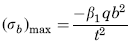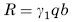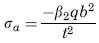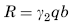Related Resources: calculators

### Loading Uniformly Decreasing Fixed Edge to Zero at free edge Equation and Calculator

Flat Rectangular Plate, Three Edges Fixed, One Edge (a) Free Loading Uniformly decreasing from fixed edge to zero at free edge Equation and Calculator.

Per. Roarks Formulas for Stress and Strain for flat plates with straight boundaries and constant thickness

Flat Rectangular plate, Three Edges Fixed, One Edge Loading Uniformly decreasing from fixed edge to zero at free edge.

 Rectangular plate; Three Edges Fixed One Edge FreeLoading Uniformly decreasing from fixed edge to zero at free edgeAt x = 0, z = 0
Stress and Reaction ForceReaction ForceStress and Reaction Force at x = ± a/2, z = b if a > b or z = 0.4b if a < bReaction ForceWhere used:
E = Modulus of Elasticity (lbs/in2)
q = Total load or force to Plate (lbs/in2)
v = Poisson’s ratio (assumed to be 0.3)
t = plate thickness, (in)
a = plate length, (in)
b = plate width, (in)
σb = stress, (lbs/in2)
R = Reaction Force (lbs/in)
β1,2 = Constant From Table A
γ1,2 = Constant From Table A

Table A

 a/b 0.25 0.5 0.75 1 1.5 2 3 β1 0.018 0.064 0.12 0.195 0.351 0.507 0.758 β2 0.019 0.068 0.125 0.166 0.244 0.387 0.514 γ1 0.106 0.195 0.265 0.324 0.406 0.458 0.505 γ2 0.075 0.151 0.211 0.242 0.106 0.199 0.313

Reference:

Roarks Formulas for Stress and Strain, 7th Edition